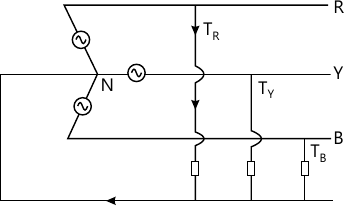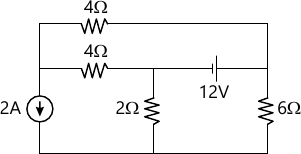MORE IN Basic Electricals & Electronics Engg.
RGPV First Year Engineering (Set B) (Semester 2)
Basic Electricals & Electronics Engg.
December 2012
Total marks: --
Total time: --
INSTRUCTIONS
(1) Assume appropriate data and state your reasons
(2) Marks are given to the right of every question
(3) Draw neat diagrams wherever necessary

Answer any two question from Q1.Q2. or Q3. Q4
1 A 400 volt, 3 phase 4 wire system supplies resistive loads between each of the three lines and neutral. Calculate the lines and neutral current when the phase sequence is RYB.7 M

2 (a) State Thevenin's Theorem giving an example.
4 M
2 (b) Using Thevenin's Theorem find the current flowing through 6? resistor of the network shown in Fig. 2.3 M

3 A balanced star connected load is supplied from a symmetrical 3 phase 400 volt (line to line) supply. The current in each phase is 50 Amp and lags 30° behind phase voltage. Find
(A) Phase voltage
(B) Phase impedance
(C) Active and reactive power drawn by the load.
Also draw the phasor diagram for the same.
7 M

4 Explain in brief the following :-
(A) Active and reactive power
(B) Apparent power
(C) Power factor
(D) Balanced and unbalanced supply
(E) Superposition theorem.
7 M

Answer any two question from Q5. Q6 Or Q7. Q.8
5 What are the assumptions made for an ideal transformer. Draw the equivalent circuit and phasor diagram of an ideal transformer.
7 M

6 An audio frequency transformer in employed to couple a 60? resistive load a source of 6 volt in series with the resistance of 2400 ?
(a) Determine the transformer turns-ratio to ensure the maximum power is transferred to the load.
(b) Calculate the value of maximum power and corresponding load current and voltage.
7 M

7 How transformer is used for impedance transformation. Explain the no load test used for the transformer parameter determination.
7 M

8 State Ampere's circuit law. What is mmf and flux density. How ampere circuital law is used in magnetic circuit analysis. Explain Hysteresis and Eddy current losses.
7 M

Answer any two question Q9. Q10 or Q11. Q12
9 Explain the constructional and operational feature of a DC machine with the help of neat diagram.
7 M

10 A three phase 440 volt, 50 hp, 50 Hz induction motor delivers rated output power at 1440 rpm. Find. (a) No. of poles of machine
(b) Synchronous speed
(C) Slip
(D) Slip rpm
(E) Rotor speed w.r.t. (i) Rotor structure (ii) Stator (iii) Stator rotating mmf.
(f) Rotor emf at operating speed if stator to rotor turn ratio is 1:0.5. Assume winding factor is unity.
7 M

11 What is the basic working principle of synchronous machine.
7 M

12 Explain Torque-slip characteristics of a 3 phase induction motor.
7 M

Answer any two question Q13. Q14 or Q15. Q16
13 State Demorgan's theorem. Specify the truth table and logic diagram for full adder circuit.
7 M

14 Draw the logic diagram for JK flip flop. Explain its operation.
7 M

15 Explain the operation of clocked RS flip flop with the help of logical diagram. Truth table. Symbol and characteristics equation.
7 M

16 Converts the following numbers into decimal
(i) (1001010.0101)2 (ii) (12212)3 (iii) (8,3)9
Also find the 2' complement of
(i) (110110)2 (ii) (10000)2
7 M

Answer any two question Q17. Q18 or Q19. Q20
17 Draw the VI characteristics of a Germanium Diode. Explain the same.
7 M

18 Why BJT's are used. Explain the working of a CB transistor.
7 M

19 Discuss the three configuration of transistor. How do they differ from each other.
7 M

20 What happens to the conductivity of the semiconductor and a metal When temperature is increased. Discuss with reason.
7 M

More question papers from Basic Electricals & Electronics Engg.